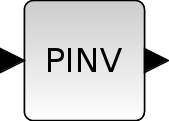Change language to:
English - Français - Português - Русский

See the recommended documentation of this function

Scilabヘルプ >> Xcos > palettes > Matrix operation palette > MATPINV

# MATPINV

Matrix PseudoInverse

### Block Screenshot### Description

The MATPINV Block outputs the inverse of a non square input matrix using the SVD theory. if the SVD decomposition of A is equal to:

A=USV'

The pseudoinverse X of A is given by:

X=VS"U' where S"(i,j)=1/S(i,j) (if S(i,j) =0), U' and V are respectively the transpose of U and V'.

and we have A*X*A=A and X*A*X=X. Both A*X and X*A are Hermitian. A warning message is printed if the input is badly scaled or nearly singular.

When the input is a MxN matrix the output is a NxM matrix.

The equivalent function of this block in Scilab is pinv.

### Parameters• Datatype(1=real double 2=Complex)

It indicates the type of the output. It support only the two types double (1) and complex (2). If we input another entry in this label xcos will print the message "Datatype is not supported".

Properties : Type 'vec' of size 1.

### Default properties

• always active: no

• direct-feedthrough: yes

• zero-crossing: no

• mode: no

• regular inputs:

- port 1 : size [-1,-2] / type 1

• regular outputs:

- port 1 : size [-2,-1] / type 1

• number/sizes of activation inputs: 0

• number/sizes of activation outputs: 0

• continuous-time state: no

• discrete-time state: no

• object discrete-time state: no

• name of computational function: mat_pinv

### Interfacing function

• SCI/modules/scicos_blocks/macros/MatrixOp/MATPINV.sci

### Computational function

• SCI/modules/scicos_blocks/src/c/mat_pinv.c
• SCI/modules/scicos_blocks/src/c/matz_pinv.c